Circuit 200 Fitness = 149.92 ( 1 inversions 7 steps 7 comparators )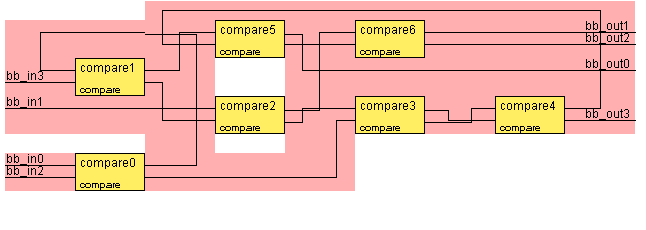Input

(4,3,2,1)

(3,4,1,2)

(3,2,1,4)

(1,3,2,4)

(2,1,4,3)

(1,4,2,3)

(2,3,4,1)

Output

(1,2,3,4)

(1,2,3,4)

(1,2,3,4)

(1,2,3,4)

(2,1,3,4)

(1,2,3,4)

(1,2,3,4)

Circuit 400 Fitness = 5.07 ( 0 inversions 5 steps 7 comparators )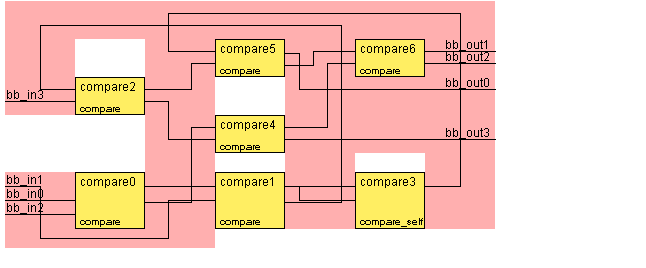Input

(4,3,2,1)

(3,4,1,2)

(3,2,1,4)

(1,3,2,4)

(2,1,4,3)

(1,4,2,3)

(2,3,4,1)

Output

(1,2,3,4)

(1,2,3,4)

(1,2,3,4)

(1,2,3,4)

(1,2,3,4)

(1,2,3,4)

(1,2,3,4)

Circuit 600 Fitness = 4.06 ( 0 inversions 4 steps 6 comparators )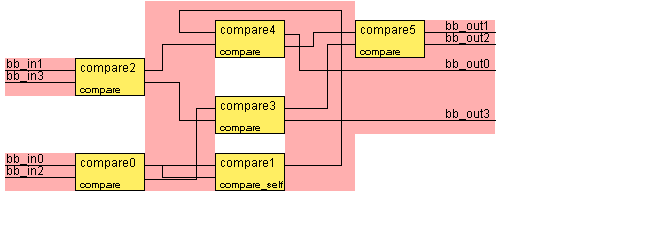Input

(4,3,2,1)

(3,4,1,2)

(3,2,1,4)

(1,3,2,4)

(2,1,4,3)

(1,4,2,3)

(2,3,4,1)

Output

(1,2,3,4)

(1,2,3,4)

(1,2,3,4)

(1,2,3,4)

(1,2,3,4)

(1,2,3,4)

(1,2,3,4)

Circuit 800 Fitness = 3.05 ( 0 inversions 3 steps 5 comparators )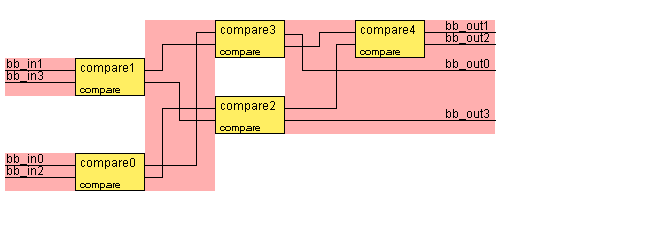Input

(4,3,2,1)

(3,4,1,2)

(3,2,1,4)

(1,3,2,4)

(2,1,4,3)

(1,4,2,3)

(2,3,4,1)

Output

(1,2,3,4)

(1,2,3,4)

(1,2,3,4)

(1,2,3,4)

(1,2,3,4)

(1,2,3,4)

(1,2,3,4)

Circuit 1000 Fitness = 3.05 ( 0 inversions 3 steps 5 comparators )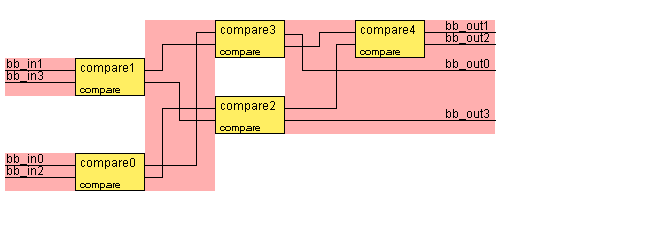Input

(4,3,2,1)

(3,4,1,2)

(3,2,1,4)

(1,3,2,4)

(2,1,4,3)

(1,4,2,3)

(2,3,4,1)

Output

(1,2,3,4)

(1,2,3,4)

(1,2,3,4)

(1,2,3,4)

(1,2,3,4)

(1,2,3,4)

(1,2,3,4)

Circuit 1200 Fitness = 3.05 ( 0 inversions 3 steps 5 comparators )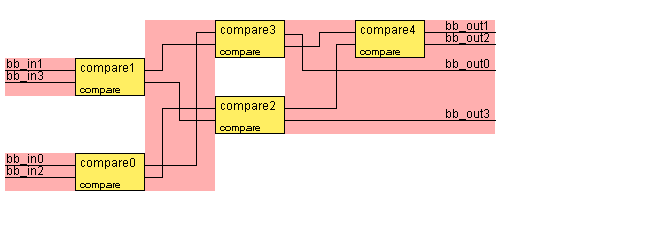Input

(4,3,2,1)

(3,4,1,2)

(3,2,1,4)

(1,3,2,4)

(2,1,4,3)

(1,4,2,3)

(2,3,4,1)

Output

(1,2,3,4)

(1,2,3,4)

(1,2,3,4)

(1,2,3,4)

(1,2,3,4)

(1,2,3,4)

(1,2,3,4)

Circuit 1400 Fitness = 3.05 ( 0 inversions 3 steps 5 comparators )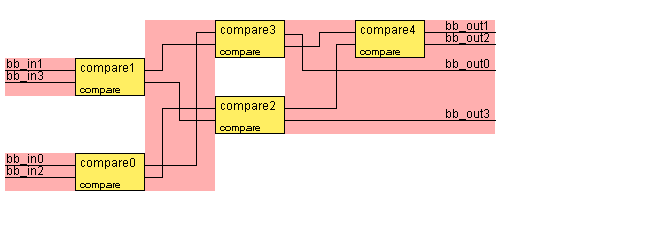Input

(4,3,2,1)

(3,4,1,2)

(3,2,1,4)

(1,3,2,4)

(2,1,4,3)

(1,4,2,3)

(2,3,4,1)

Output

(1,2,3,4)

(1,2,3,4)

(1,2,3,4)

(1,2,3,4)

(1,2,3,4)

(1,2,3,4)

(1,2,3,4)

Circuit 1600 Fitness = 3.05 ( 0 inversions 3 steps 5 comparators )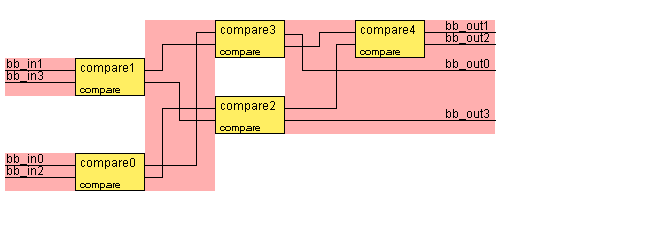Input

(4,3,2,1)

(3,4,1,2)

(3,2,1,4)

(1,3,2,4)

(2,1,4,3)

(1,4,2,3)

(2,3,4,1)

Output

(1,2,3,4)

(1,2,3,4)

(1,2,3,4)

(1,2,3,4)

(1,2,3,4)

(1,2,3,4)

(1,2,3,4)

Circuit 1800 Fitness = 3.05 ( 0 inversions 3 steps 5 comparators )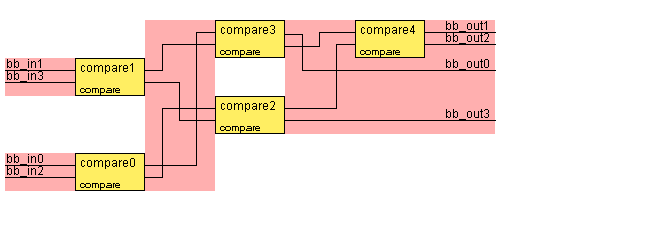Input

(4,3,2,1)

(3,4,1,2)

(3,2,1,4)

(1,3,2,4)

(2,1,4,3)

(1,4,2,3)

(2,3,4,1)

Output

(1,2,3,4)

(1,2,3,4)

(1,2,3,4)

(1,2,3,4)

(1,2,3,4)

(1,2,3,4)

(1,2,3,4)

Circuit 2000 Fitness = 3.05 ( 0 inversions 3 steps 5 comparators )Input

(4,3,2,1)

(3,4,1,2)

(3,2,1,4)

(1,3,2,4)

(2,1,4,3)

(1,4,2,3)

(2,3,4,1)

Output

(1,2,3,4)

(1,2,3,4)

(1,2,3,4)

(1,2,3,4)

(1,2,3,4)

(1,2,3,4)

(1,2,3,4)